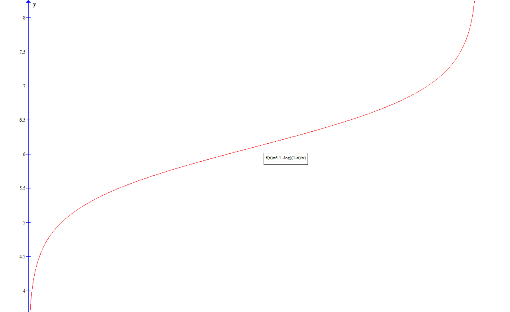#### You may also like### Very Old Man

Is the age of this very old man statistically believable?### bioNRICH

bioNRICH is the area of the stemNRICH site devoted to the mathematics underlying the study of the biological sciences, designed to help develop the mathematics required to get the most from your study of biology at A-level and university.### Catalyse That!

Can you work out how to produce the right amount of chemical in a temperature-dependent reaction?

# Blood Buffers

##### Age 16 to 18 Challenge Level:

At a glance this initial part of the question appears exceptionally tricky. However, by breaking the simultaneous equilibrium into two separate equilibria, the problem is actually reasonably easy to analyse.

The first of these equilibria is:

$$H^+ + HCO_3^- + H_2O \rightleftharpoons^{K_1} H_2CO_3 + H_2O$$

From the original definition of K, it can be seen that:

$$K_1 = \frac{[H_2CO_3]}{[H^+][HCO_3^-]}$$

The second equilibrium is:

$$H_2CO_3 + H_2O \rightleftharpoons^{K_2} 2H_2O + CO_2$$

Again, from the definition of K, we can easily see that:

$$K_2 = \frac{[CO_2]}{[H_2CO_3]}$$

Eliminating $[H_2CO_3]$ from these gives:

$$K_1[H^+][HCO_3^-] = \frac{[CO_2]}{K_2}$$

Rearranging:

$$[H^+] = \frac{[CO_2]}{K_1K_2[HCO_3^-]}$$

Using the definition of pH allows:

$$pH = -log_{10}\left(\frac{[CO_2]}{K_1K_2[HCO_3^-]}\right)$$
$$= -log_{10}\left(\frac{1}{K_1K_2}\right) -log_{10}\left(\frac{[CO_2]}{[HCO_3^-]}\right)$$
$$= -log_{10}\left(\frac{1}{K}\right) -log_{10}\left(\frac{[CO_2]}{[HCO_3]}\right)$$
$$= pK -log\left(\frac{[CO_2]}{[HCO_3]}\right)$$

For the second part of this question, we are trying to prove that the inside of the logarithm is equivalent in each equation. Starting with the final equation and working in reverse:

$\frac{1}{x} -1 -K_1[H^+]$

$= \left(\frac{[HCO_3^-] + [H_2CO_3] + [CO_2]}{[HCO_3^-]}\right)\ -1\ -\left(\frac{[H^+][H_2CO_3]}{[H^+][HCO_3^-]}\right)$

$= \left(\frac{[H_2CO_3]}{[HCO_3^-]}\right) + \left(\frac{[CO_2]}{[HCO_3^-]}\right) - \left(\frac{[H_2CO_3]}{[HCO_3^-]}\right)$

$= \left(\frac{[CO_2]}{[HCO_3^-]}\right)$

This is as required.

By treating $K_1[H^+]$ as very small, we can neglect it, and as the pH expression becomes:

$$pH = 6.1 - log( \frac{1}{x} -1)$$
$$= 6.1 - log\left(\frac{1-x}{x}\right)$$

Plotting this gives the required buffer curve:Extension:

1) We are dealing with pHs in the region of 4- 8, and so the $[H^+] = 10^{-4} to 10^{-8}$. This is very small compared to the $\frac{1}{x} -1$ term, and so can be neglected. Additionally, because this quantity is subsequently logged, such a small difference is made even smaller, such that it can certainly be neglected.

2) This part of the problem requires a solid grip on the chain rule, and logarithmic manipulation. It is solved as shown:

$$pH = 6.1 - log\left(\frac{1-x}{x}\right)$$
$$= 6.1 - \frac{ln\left(\frac{1-x}{x}\right)}{ln(10)}$$
$$= 6.1 - \frac{1}{ln(10)}\left(ln(1-x) -ln(x)\right)$$

$$\therefore \frac{d(pH)}{dx}= \frac{1}{ln(10)} \left(\frac{1}{1-x} + \frac{1}{x}\right)$$

$$\therefore \frac{d^2(pH)}{dx^2} = \frac{1}{ln(10)} \left(\frac{1}{(1-x)^2} -\frac{1}{x^2}\right) = 0$$

$$\frac{1}{(1-x)^2} = \frac{1}{x^2}$$
$$x =0.5$$

Thus the second derivative of the pH is zero when x = 0.5. This corresponds to a point of inflection on the graph, which is where the gradient of the gradent is zero: at this point the curve ceases to decrease in gradient and begins to again increase in gradient.